# Chapter 5 Population structure

## 5.1 Principal Component Analysis (PCA)

PCA on the genotype matrix can be used to capture population structure. However, PCA can actually capture different kinds of structure :

• population structure (what we want),
• LD structure, when there are two many correlated variants (e.g. within long-range LD regions) and not enough population structure (see this vignette),
• relatedness structure, when there are related individuals who usually cluster together in later PCs,
• noise, basically just circles when looking at PC scores.

Population structure is the second main topic of my research work (after polygenic scores).

• In Privé et al. (2018), I introduced an algorithm to compute PCA for a bigSNP object while accounting for the LD problem by using clumping (not pruning, cf. this vignette) and an automatic detection and removal of long-range LD regions.

• In Privé, Luu, Vilhjálmsson, et al. (2020), I improved the implementation of an algorithm, pcadapt, to detect variants associated with population structure (could be considered as a GWAS for population structure).

• In Privé, Luu, Blum, et al. (2020), I extended {bigsnpr} to also be able to run PCA on PLINK bed files directly with a small percentage of missing values, and investigated best practices for PCA in more detail.

• In Privé, Aschard, et al. (2022) and Privé (2022), I showed how to use PCA for ancestry inference, including grouping individuals in homogeneous ancestry groups, and inferring ancestry proportions from allele frequencies only (see this vignette).

## 5.2 Example

There can be many steps to properly perform a PCA, which I try to showcase in the following example. You can find another detailed example of how to conduct a PCA in this vignette.

Let us reuse the data prepared in 4.3.

First, let us get an idea of the relatedness in the data using

library(bigsnpr)
#> Loading required package: bigstatsr
(NCORES <- nb_cores())
thr.king = 2^-4.5, make.bed = FALSE, ncores = NCORES)
hist(log2(rel$KINSHIP), "FD"); abline(v = c(-4.5, -3.5), col = "red")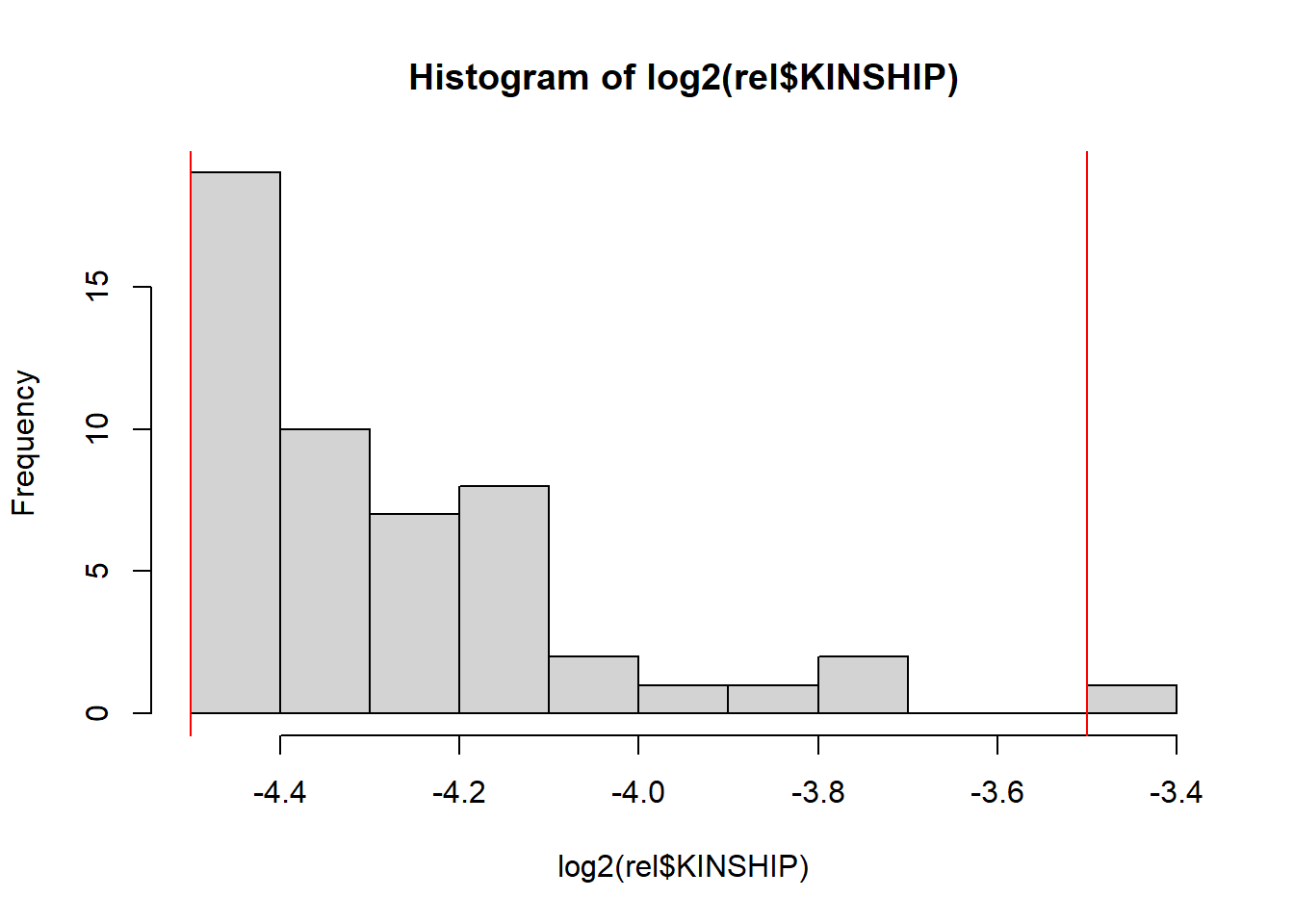When computing relatedness with KING, LD pruning is NOT recommended. However, it may be useful to filter out some variants that are highly associated with population structure, e.g. as performed in the UK Biobank . For example, see this code. Relatedness should not be a major issue here. Let us now compute PCs. All the code that follows could be run on the bigSNP object we made before. Nevertheless, to showcase the bed_* functions here, we will run the following analyses on the bed file directly. # Memory-map a bed file directly obj.bed <- bed("tmp-data/GWAS_data_sorted_QC.bed") str(obj.bed, max.level = 2) #> Reference class 'bed' [package "bigsnpr"] with 13 fields #>$ bedfile: chr "tmp-data/GWAS_data_sorted_QC.bed"
#>  $extptr :<externalptr> #>$ .fam   :'data.frame': 1401 obs. of  6 variables:
#>  $.map :'data.frame': 404663 obs. of 6 variables: #>$ prefix : chr "tmp-data/GWAS_data_sorted_QC"
#>  $bimfile: chr "tmp-data/GWAS_data_sorted_QC.bim" #>$ famfile: chr "tmp-data/GWAS_data_sorted_QC.fam"
#>  $fam :'data.frame': 1401 obs. of 6 variables: #>$ map    :'data.frame': 404663 obs. of  6 variables:
#>  $nrow : int 1401 #>$ ncol   : int 404663
#>  $light :Reference class 'bed_light' [package "bigsnpr"] with 5 fields #> ..and 15 methods, of which 1 is possibly relevant #>$ address:<externalptr>
#>  and 16 methods, of which 2 are  possibly relevant:
#>    initialize, show#envRefClass
# If you expect individuals to all come from the same continent,
# use e.g. k=10, otherwise you can try k=25.
obj.svd <- runonce::save_run(
bed_autoSVD(obj.bed, k = 12, ncores = NCORES),
file = "tmp-data/PCA_GWAS_data.rds")
#>
#> Phase of clumping (on MAC) at r^2 > 0.2.. keep 115258 variants.
#> Discarding 0 variant with MAC < 10.
#>
#> Iteration 1:
#> Computing SVD..
#> 309 outlier variants detected..
#> 2 long-range LD regions detected..
#>
#> Iteration 2:
#> Computing SVD..
#> 19 outlier variants detected..
#> 0 long-range LD region detected..
#>
#> Iteration 3:
#> Computing SVD..
#> 3 outlier variants detected..
#> 0 long-range LD region detected..
#>
#> Iteration 4:
#> Computing SVD..
#> 15 outlier variants detected..
#> 0 long-range LD region detected..
#>
#> Iteration 5:
#> Computing SVD..
#> 0 outlier variant detected..
#>
#> Converged!
#>    user  system elapsed
#>   26.58    1.34  139.57
plot(obj.svd)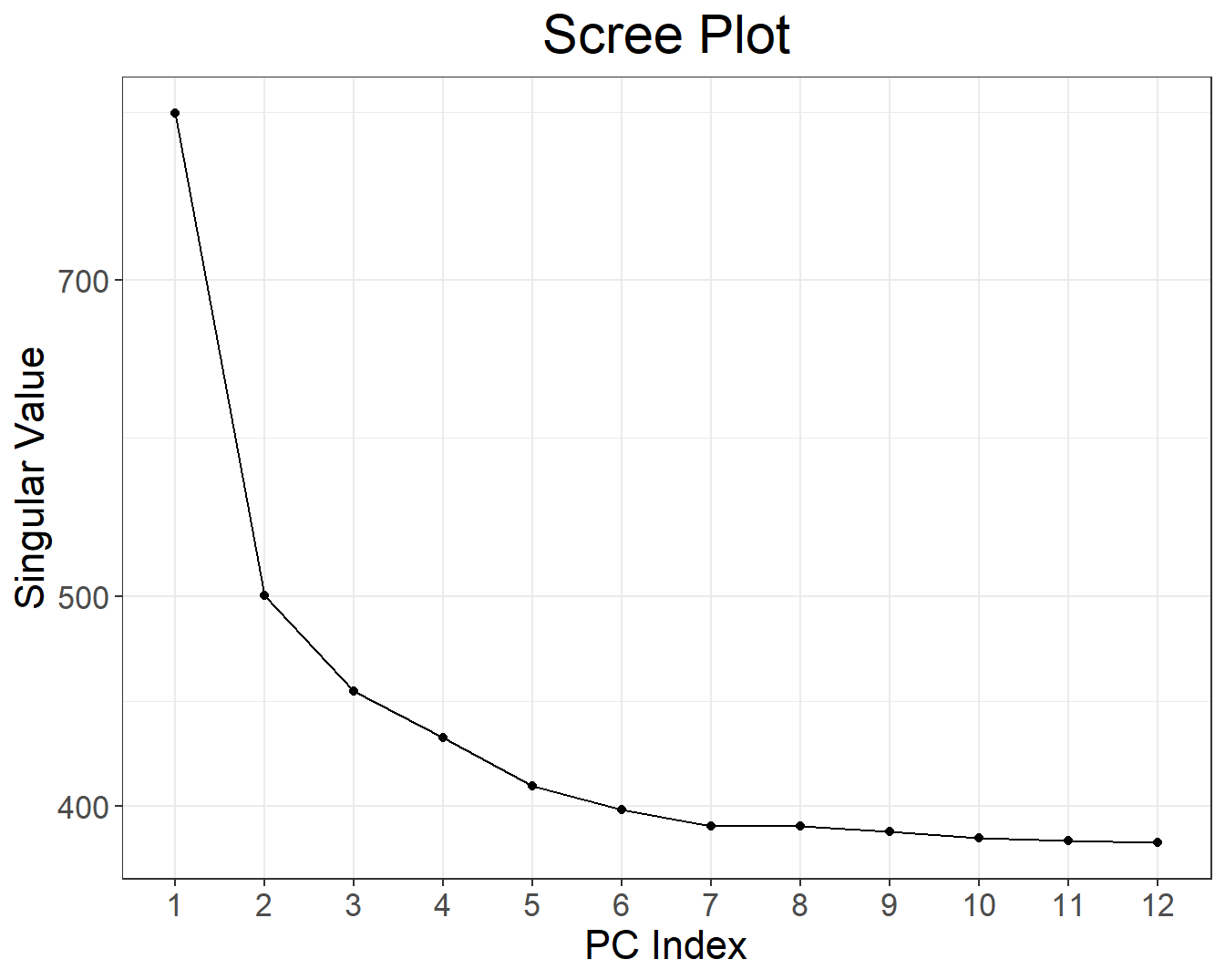plot(obj.svd, type = "scores", scores = 1:12, coeff = 0.5)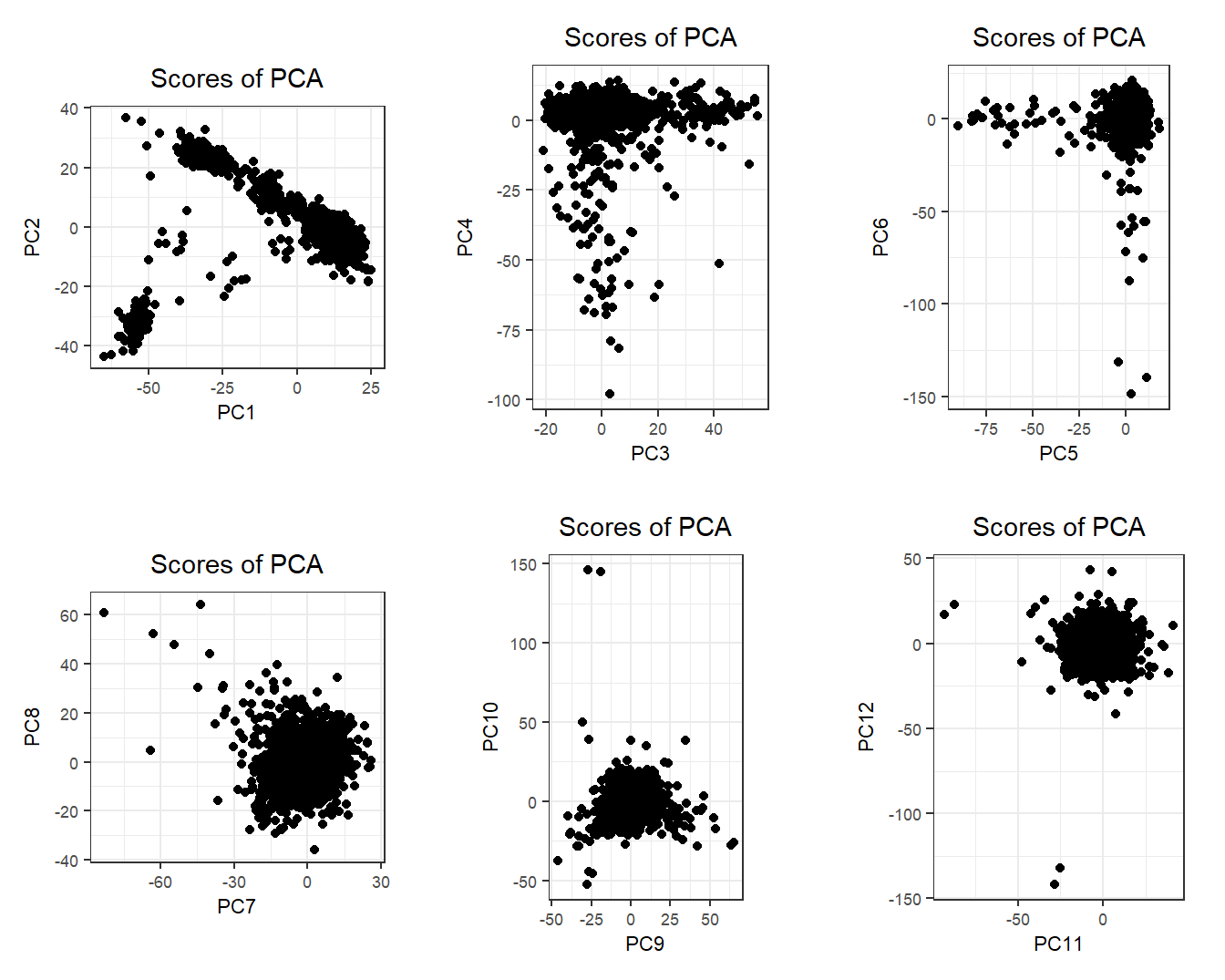There is some population structure (maybe up to 6 PCs). You should also check loadings to make sure there is no LD structure (peaks on loadings):

plot(obj.svd, type = "loadings", loadings = 1:6, coeff = 0.5)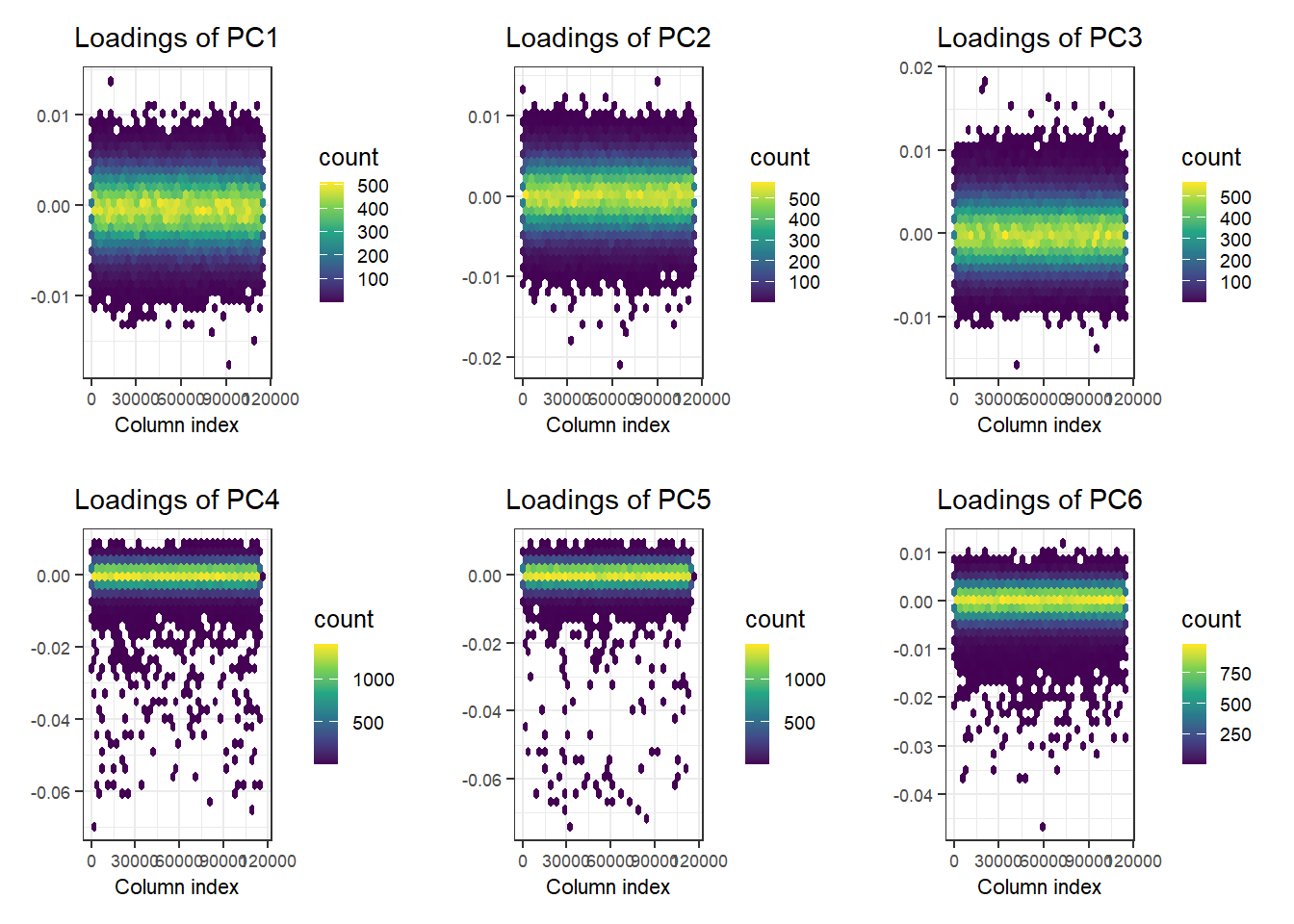No peaks, but loadings of PC4 and PC5 are a bit odd.

If you expect the individuals to mostly come from one population (e.g. in a national biobank), you can simply use a robust distance to identify a homogeneous subset of individuals, then look at the histogram of log-distances to choose a threshold based on visual inspection (here I would probably choose 4.5).

PC <- predict(obj.svd)
ldist <- log(bigutilsr::dist_ogk(PC))
hist(ldist, "FD")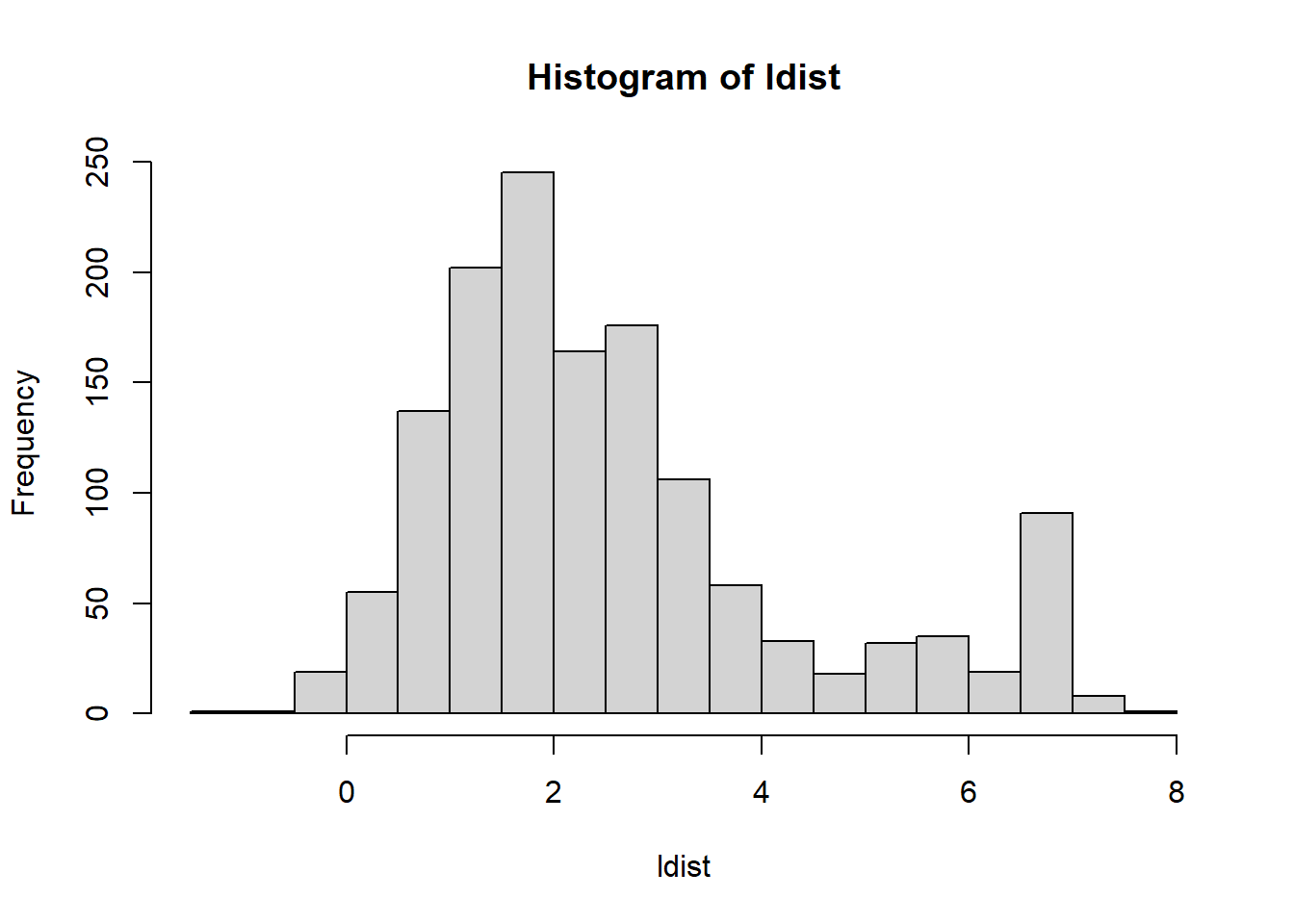library(ggplot2)
devtools::source_url(
"https://raw.githubusercontent.com/privefl/paper4-bedpca/master/code/plot_grid2.R")

plot_grid2(plotlist = lapply(1:4, function(k) {
k1 <- 2 * k - 1
k2 <- 2 * k
qplot(PC[, k1], PC[, k2], color = ldist, size = I(2)) +
scale_color_viridis_c() +
theme_bigstatsr(0.6) +
labs(x = paste0("PC", k1), y = paste0("PC", k2), color = "log-distance") +
coord_equal()
}), nrow = 2, legend_ratio = 0.2, title_ratio = 0)
#> Warning: qplot() was deprecated in ggplot2 3.4.0.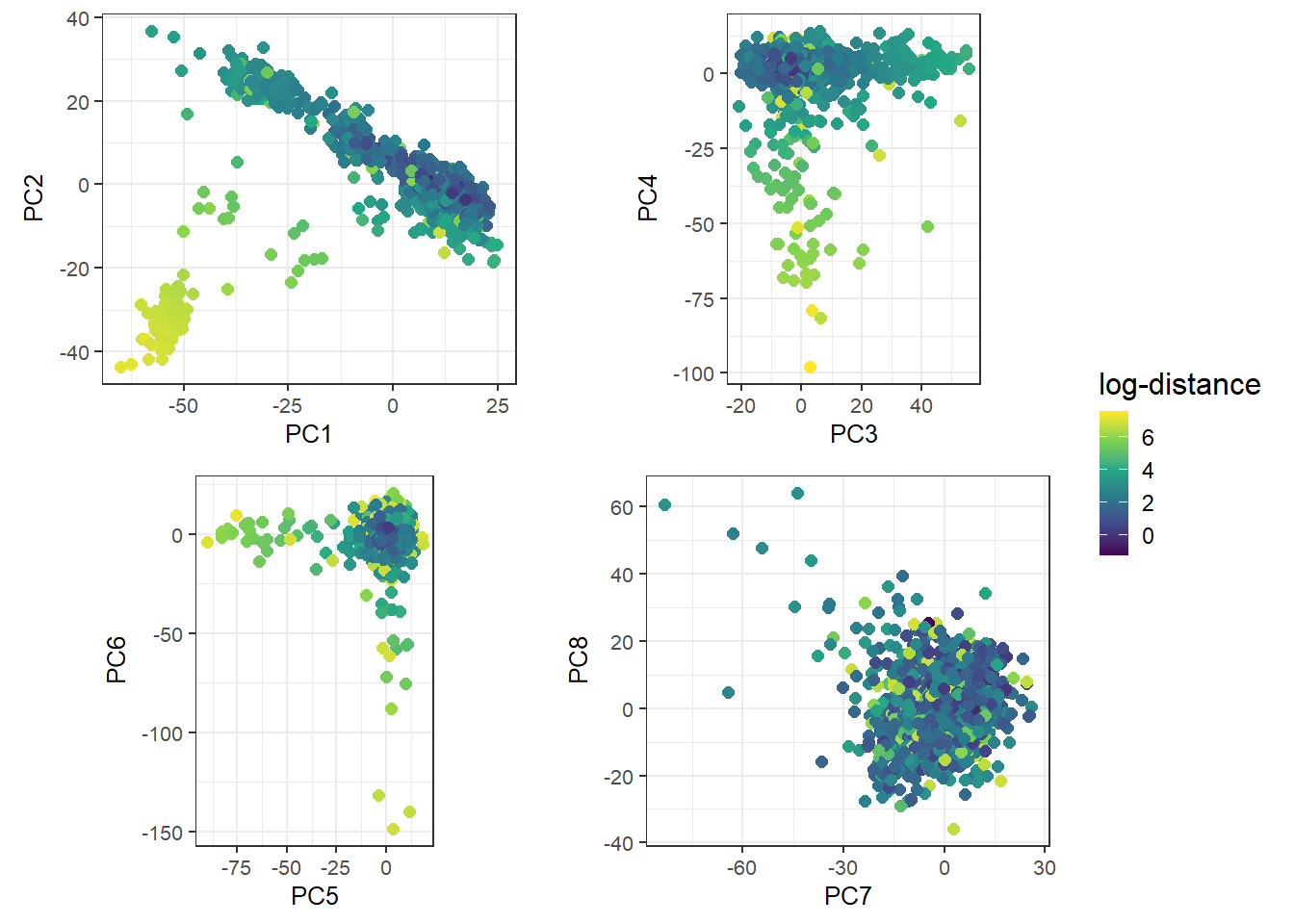It would be nice if we could get a better sense of the ancestry of these individuals. To achieve this, we can project this data onto the PCA space of many known population groups defined in Privé (2022).

all_freq <- bigreadr::fread2(
dir = "tmp-data", fname = "ref_freqs.csv.gz"))

dir = "tmp-data", fname = "projection.csv.gz"))

# coefficients to correct for overfitting of PCA
correction <- c(1, 1, 1, 1.008, 1.021, 1.034, 1.052, 1.074, 1.099,
1.123, 1.15, 1.195, 1.256, 1.321, 1.382, 1.443)
library(dplyr)
matched <- obj.bed$map %>% transmute(chr = chromosome, pos = physical.pos, a1 = allele1, a0 = allele2) %>% mutate(beta = 1) %>% snp_match(all_freq[1:5]) %>% print() #> chr pos a0 a1 beta _NUM_ID_.ss rsid _NUM_ID_ #> 1 1 752566 G A -1 2 rs3094315 1 #> 2 1 785989 T C -1 4 rs2980300 2 #> 3 1 798959 G A 1 5 rs11240777 3 #> 4 1 947034 G A -1 6 rs2465126 4 #> 5 1 949608 G A 1 7 rs1921 5 #> 6 1 1018704 A G -1 8 rs9442372 6 #> [ reached 'max' / getOption("max.print") -- omitted 301150 rows ] # further subsetting on missing values counts <- bed_counts(obj.bed, ind.col = matched$_NUM_ID_.ss, ncores = NCORES)
# hist(nb_na <- counts[4, ])
ind <- which(counts[4, ] < (nrow(obj.bed) * 0.05))

# project individuals (divided by 2) onto the PC space
PROJ <- as.matrix(projection[matched$_NUM_ID_[ind], -(1:5)]) all_proj <- apply(sweep(PROJ, 2, correction / 2, '*'), 2, function(x) { bed_prodVec(obj.bed, x, ind.col = matched$_NUM_ID_.ss[ind], ncores = NCORES,
# scaling to get G if beta = 1 and (2 - G) if beta = -1
center = 1 - matched$beta[ind], scale = matched$beta[ind])
})

all_centers <- crossprod(as.matrix(all_freq[matched\$_NUM_ID_[ind], -(1:5)]), PROJ)
all_sq_dist <- apply(all_centers, 1, function(one_center) {
rowSums(sweep(all_proj, 2, one_center, '-')^2)
})

THR <- 0.002  # you can adjust this threshold
thr_sq_dist <- max(dist(all_centers)^2) * THR / 0.16

group <- colnames(all_freq)[-(1:5)]
group[group %in% c("Scandinavia", "United Kingdom", "Ireland")]   <- "Europe (North West)"
group[group %in% c("Europe (South East)", "Europe (North East)")] <- "Europe (East)"

cluster <- apply(all_sq_dist, 1, function(sq_dist) {
ind <- which.min(sq_dist)
if (sq_dist[ind] < thr_sq_dist) group[ind] else NA
})

table(cluster, exclude = NULL)  # no NA -> all assigned
#> cluster
#>           Ashkenazi       Europe (East) Europe (North West)
#>                 110                 148                 872
#> Europe (South West)               Italy         Middle East
#>                  45                 219                   4
#>                <NA>
#>                   3
plot_grid2(plotlist = lapply(1:4, function(k) {
k1 <- 2 * k - 1
k2 <- 2 * k
qplot(PC[, k1], PC[, k2], color = cluster, size = I(2)) +
theme_bigstatsr(0.6) +
labs(x = paste0("PC", k1), y = paste0("PC", k2), color = "Assigned\npopulation") +
coord_equal()
}), nrow = 2, legend_ratio = 0.25, title_ratio = 0)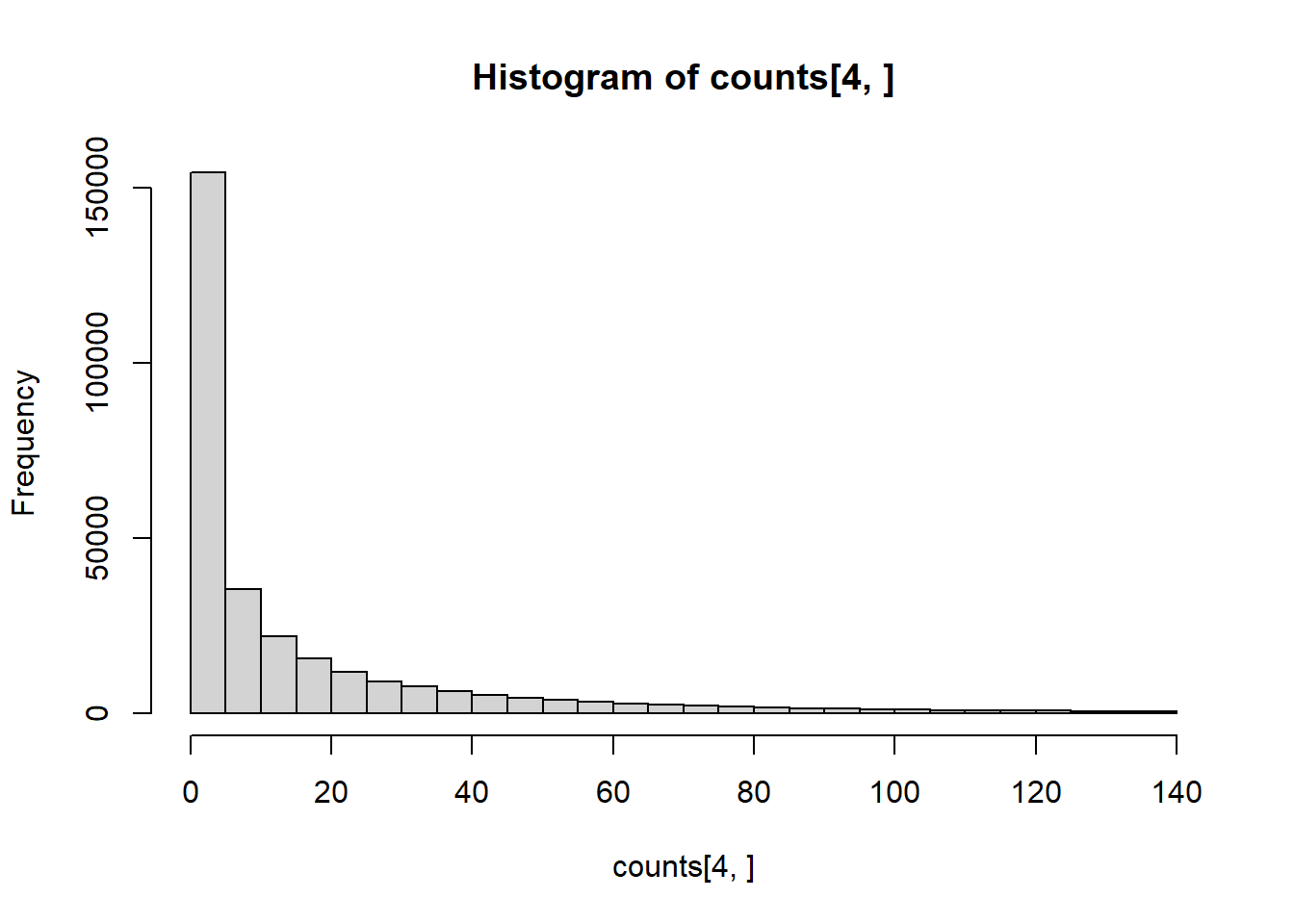These are mostly European individuals. PC4-PC6 are definitively a bit odd.

Adapt all the previous code to rerun it on the bigSNP object we made before (instead of the bed object we have used here).

### References

Bycroft, C., Freeman, C., Petkova, D., Band, G., Elliott, L.T., Sharp, K., et al.others. (2018). The UK Biobank resource with deep phenotyping and genomic data. Nature, 562, 203–209.
Privé, F. (2022). Using the UK Biobank as a global reference of worldwide populations: application to measuring ancestry diversity from GWAS summary statistics. Bioinformatics, 38, 3477–3480.
Privé, F., Aschard, H., Carmi, S., Folkersen, L., Hoggart, C., O’Reilly, P.F., & Vilhjálmsson, B.J. (2022). Portability of 245 polygenic scores when derived from the UK Biobank and applied to 9 ancestry groups from the same cohort. The American Journal of Human Genetics, 109, 12–23.
Privé, F., Aschard, H., Ziyatdinov, A., & Blum, M.G.B. (2018). Efficient analysis of large-scale genome-wide data with two R packages: bigstatsr and bigsnpr. Bioinformatics, 34, 2781–2787.
Privé, F., Luu, K., Blum, M.G., McGrath, J.J., & Vilhjálmsson, B.J. (2020). Efficient toolkit implementing best practices for principal component analysis of population genetic data. Bioinformatics, 36, 4449–4457.
Privé, F., Luu, K., Vilhjálmsson, B.J., & Blum, M.G. (2020). Performing highly efficient genome scans for local adaptation with R package pcadapt version 4. Molecular Biology and Evolution, 37, 2153–2154.